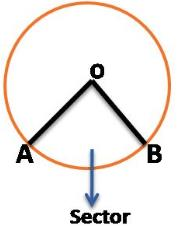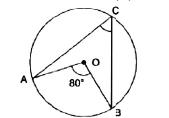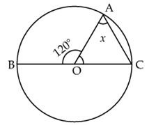Courses

# Test: Introduction To Circles

## 10 Questions MCQ Test Mathematics (Maths) Class 9 | Test: Introduction To Circles

Description
This mock test of Test: Introduction To Circles for Class 9 helps you for every Class 9 entrance exam. This contains 10 Multiple Choice Questions for Class 9 Test: Introduction To Circles (mcq) to study with solutions a complete question bank. The solved questions answers in this Test: Introduction To Circles quiz give you a good mix of easy questions and tough questions. Class 9 students definitely take this Test: Introduction To Circles exercise for a better result in the exam. You can find other Test: Introduction To Circles extra questions, long questions & short questions for Class 9 on EduRev as well by searching above.
QUESTION: 1

### The region between an arc and the two radii joining the centre of the end points of the arc is called a:

Solution:Sector is the region between an arc and two radii joining the center to the end points of the arc. For example, in the given figure, OAB is the sector of the circle.

QUESTION: 2

### Circles having the same centre and different radii are called

Solution:Concentric circles are circles with a common center. The region between two concentric circles of different radii is called an annulus. Any two circles can be made concentric by inversion by picking the inversion center as one of the limiting points.

QUESTION: 3

### In the figure, if O is the centre of a circle, then the measure of ∠ACB is:Solution:

The angle subtented by the arc at the centre is double the angle subtented by it at any point on the circle

QUESTION: 4

A circle divides the plane in which it lies into

Solution:

Circle dividing the plane: Circle divides the plane on which it lies into three parts. They are:
- inside the circle, which is also called the interior of the circle;
- the circle and
- Outside the circle, this is also called the exterior of the circle.
The circle and its interior make up the circular region.

QUESTION: 5

Segment of a circle is the region between an arc and ………..of the circle.

Solution:

Segment of a Circle
The region between a chord of a circle and its associated arc.

QUESTION: 6

A chord of a circle which is twice as long as its radius is a … of the circle

Solution:

Diameter is the longest chord of a circle and is always twice the radius.
[∵ Diameter = 2 × Radius]

QUESTION: 7

The degree measure of a semicircle is

Solution:

One circle measures 360 degree.the half of circle is called semi-circle.thus,measure of semi-circle=360/2=180 degree.

QUESTION: 8

A line that intersects a circle in two distinct points is a

Solution:

A secant of a curve is a line that intersects the curve in at least two (distinct) points.The word secant comes from the Latin word secare, meaning to cut. In the case of a circle, a secant will intersect the circle in exactly two points and a chord is the line segment determined by these two points, that is the interval on a secant whose endpoints are these points.

QUESTION: 9

In the figure, if O is the centre of the circle and ∠BOA = 120°, then the value of x isSolution:

∠BOA = 120 (given)

∠AOC = 60 (LINEAR PAIR )

In triangle OAC,

OA = OC (Radii of same circle )

∠OAC =∠OCA = x

(angle opposite to equal sides are equal )

∠A + ∠C +∠O = 180

x + x + 60 = 180

2x = 120

x =60o

QUESTION: 10

The longest chord of the circle is the

Solution:

A chord is a line segment which joins two points on the circumference of a circle, and the longest chord of a circle is the diameter of the circle since the diameterpasses through and contains the center of the circle; The longest distance across a circle is through its center.